## Wednesday, November 17, 2010

### Examining PMML 4.0: Pre-Processing and Data Manipulation

You may be wondering what is all the fuss around PMML and its 4.0 version. So, we decided to explore all that PMML 4.0 has to offer in a series of blogs. In part I, we will be exploring its improved pre-processing capabilities.

All data mining models manipulate the raw data in a way or another before passing it through a neural network, support vector machine, or regression model. Therefore, a language that wants to represent all the computations that go into a model needs also to be able to represent the data transformations that were applied to the raw data before scoring takes place. PMML is this language! It is the Yin and Yang of data mining.Let's first re-cap on the pre-processing capabilities available in PMML 3.2. This version of PMML allows for the following out of the box data transformations:
• Normalization of continuous variables: this is accomplished via the NormContinuous element of PMML. It is mostly used to normalized a variable between 0 and 1. See example below (real PMML code) in which two variables are normalized. The first between 0 and 1 and the second between 0 and 4.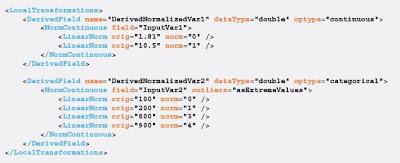• Normalizing Categorical Inputs: normally used to transform strings into numerical variables. This is accomplished by the element NormDiscrete. In the PMML example below, a categorical variable creates dummy variables that will be assigned values 1 or 0 depending on the category assumed by the input variable.• Value Mapping: this is accomplished in PMML by the use of a mapping table and the element MapValues. To make things more interesting, in the PMML example below, we combine elements MapValues and NormDiscrete to group small sets of categorical values. In specific, we want to find out if the input variable belongs to a specific group of colors. We do that by using MapValues to map different colors to the same number. We then use the element NormDiscrete to create dummy variables which are used to indicate group membership.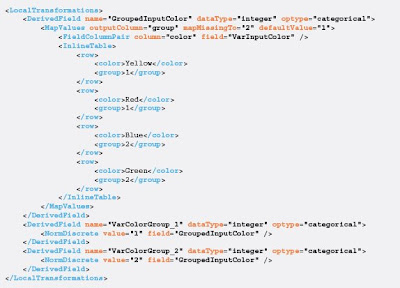• Arithmetic Expressions: PMML offers a range of arithmetic functions (as well as string and date/time maniputation functions) that can be arranged in different ways to express complex arithmetic expressions. The example below solves the following operation:
• PMML 4.0 - Boolean Operations: Not only PMML 4.0 allows for Boolean operations to be fully expressed, but it also allows these to be nested into IF-THEN-ELSE logic. These new buit-in functions offer a vast new array of possibilites for representing data transformations in PMML. So, we devote the rest of this review by looking at transformations that can now be easily expressed in PMML 4.0.
We start with the PMML code below which implements the following logical and arithmetic operations:
IF InputVar1 == "Partner" THEN DerivedVar1 = "P" ELSE DerivedVar2 = 2 * InputVar2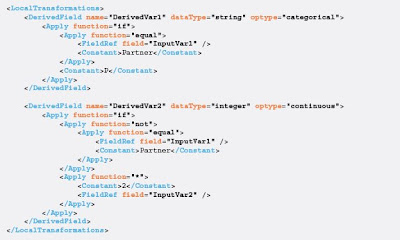Note that it uses the newly defined 4.0 functions: "if", "equal", and "not" as well as function "*".

The PMML code below assumes that both "then" and "else" parts of the "if" use the same derived variable to implement the following operations:
IF InputVar1 == "Partner" THEN DerivedVar1 = "5.1 * InputVar2" ELSE DerivedVar1 = "InputVar2 / 3.3"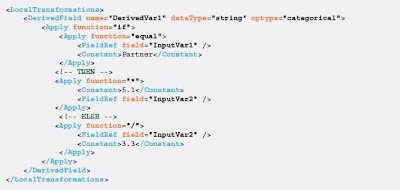Finally, we end our list of PMML pre-processing examples by showing the use of 4.0 functions "isMissing" and "isIn" combined with function "if". The PMML example below implements the following operations:
IF InputVar is missing THEN DerivedVar = 1 ELSE (IF InputVar is in ("Partner", "Associate", "Colleague") THEN DerivedVar = 2 ELSE DerivedVar = 3)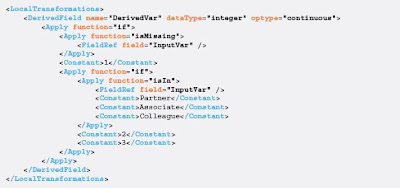We finish part I of our PMML tour hoping that this short description of its pre-processing capabilities can help you to easily navigate through all the data transformations available in PMML 4.0.